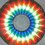# Yet another proof note.

Prove that highest power of 2 in $2n!$ is strictly greater than the highest power of 2 in $n!^2$.

This is kind of a corollary from another fundamental theorem. If you know that theorem, then this problem is nothing.

And this one is original to the best of my knowledge. I got it from the theorem.Note by Vishnu C
6 years, 1 month ago

This discussion board is a place to discuss our Daily Challenges and the math and science related to those challenges. Explanations are more than just a solution — they should explain the steps and thinking strategies that you used to obtain the solution. Comments should further the discussion of math and science.

When posting on Brilliant:

• Use the emojis to react to an explanation, whether you're congratulating a job well done , or just really confused .
• Ask specific questions about the challenge or the steps in somebody's explanation. Well-posed questions can add a lot to the discussion, but posting "I don't understand!" doesn't help anyone.
• Try to contribute something new to the discussion, whether it is an extension, generalization or other idea related to the challenge.
• Stay on topic — we're all here to learn more about math and science, not to hear about your favorite get-rich-quick scheme or current world events.

MarkdownAppears as
*italics* or _italics_ italics
**bold** or __bold__ bold
- bulleted- list
• bulleted
• list
1. numbered2. list
1. numbered
2. list
Note: you must add a full line of space before and after lists for them to show up correctly
paragraph 1paragraph 2

paragraph 1

paragraph 2

[example link](https://brilliant.org)example link
> This is a quote
This is a quote
    # I indented these lines
# 4 spaces, and now they show
# up as a code block.

print "hello world"
# I indented these lines
# 4 spaces, and now they show
# up as a code block.

print "hello world"
MathAppears as
Remember to wrap math in $$ ... $$ or $ ... $ to ensure proper formatting.
2 \times 3 $2 \times 3$
2^{34} $2^{34}$
a_{i-1} $a_{i-1}$
\frac{2}{3} $\frac{2}{3}$
\sqrt{2} $\sqrt{2}$
\sum_{i=1}^3 $\sum_{i=1}^3$
\sin \theta $\sin \theta$
\boxed{123} $\boxed{123}$

## Comments

Sort by:

Top Newest

Hint:

The theorem is this: $\left(^{2n} _n\right)$ is always even. From this theorem, this corollary is pretty easy to arrive at. But can you prove this theorem?

- 6 years, 1 month ago

Log in to reply

Here's how you prove that $(^{2n}_n)$ is even:

$(^{n}_r)=(^{n-1}_r)+(^{n-1}_{r-1})$

Put n=2n, r=n and you get:

$(^{2n}_n)=(^{2n-1}_{n-1})+(^{2n-1}_n)\quad and\quad (^{2n-1}_n)=(^{2n-1}_{n-1})$

- 6 years, 1 month ago

Log in to reply

There is an easy way to prove the statement directly, though it essentially uses the same ideas.

Hint: $\lfloor \frac{ 2n}{2^l} \rfloor \geq 2 \lfloor \frac{n}{2^l} \rfloor$. Hint: There exists $k$ such that $n < 2^k \leq 2n$.

Staff - 6 years, 1 month ago

Log in to reply

Nice to know other direct methods :-) But I kinda developed the question from the theorem and it seemed to have an if and only if correspondence, so I decided to post it here.

I just got back from the exams and I've got many problems that I'm going to be posting here. It was really fun to solve all those challenging problems. And thanks to @Otto Bretscher 's problem, one more of those, I got the answer to a sub question pretty quickly. It was pretty much the same question : D

- 6 years, 1 month ago

Log in to reply

I look forward to seeing more of your problems!

Yay to solving hard problems via the "done before" method!

Staff - 6 years, 1 month ago

Log in to reply

Which theorem are u talking about ? that 2nCn is even? If thats the case we see :

Let us partition a set of 2n objects into 2 equal groups.We can definitely do such(e.g,a(1),a(2),...,a(n) in one group and others in the other.)----------------------- Let the number of ways of doing this be x(n).-------------------------- By definition x(n) is an integer. ----------------------From each of these partitions we get 2 unique ""n-combination of 2n objects"". -----------------------Conversely every ""n-combination of 2n objects"" paired with its conterpart gives one unique partition. -------------------So we form a 1-2 bijection between these 2 sets(the first of partitions and second of combinations). ----------------thus, by bijection principle ----------------------2nCn=2*x(n)=even.

- 6 years, 1 month ago

Log in to reply

Nice! The logical version of what I posted. I've always found it hard to imagine scenarios in combinatorics where you get the results of what you want through the implications of the scenario. That's why I try to do things through mathematical calculation first and why I find combinatorics hard. But I always like reading solutions that are made in the way you did it : )

- 6 years, 1 month ago

Log in to reply

## Though i am begining to sense a bijection to prove this but really this is not a trivial result!!!!!!!!!!!

- 6 years, 1 month ago

Log in to reply

there is another elegant way by counting the number of ways of reaching the point (2n,n) in the pascal triangle this gives (try and see by breaking the path at the nth line)------------ 2nCn=---- sumi=0 to n [(nCi)^{2}] -------------if n is odd the (nCi)^{2} and (nCn-i)^{2} can be grouped and so sum is even.--------------- if n is even all other terms except nC(n/2) are grouped and their sum becomes even.--------------- For nC(n/2), simply , we use induction hypothesis!!!!(strong form of mathematical induction)

- 6 years, 1 month ago

Log in to reply

Is this solution Okay? Its sort of extention of the pascal triangle proof

- 6 years, 1 month ago

Log in to reply

×

Problem Loading...

Note Loading...

Set Loading...